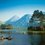# Proof of $\zeta(4) = \frac{\pi^4}{90}$

Consider the function $f(t):=t^2\ \ (-\pi\leq t\leq \pi)$, extended to all of ${\mathbb R}$ periodically with period $2\pi$. Developping $f$ into a Fourier series we get $t^2 ={\pi^2\over3}+\sum_{k=1}^\infty {4(-1)^k\over k^2}\cos(kt)\qquad(-\pi\leq t\leq \pi).$ If we put $t:=\pi$ here we easily find $\zeta(2)={\pi^2\over6}$. For $\zeta(4)$ we use Parseval's formula $\|f\|^2=\sum_{k=-\infty}^\infty |c_k|^2$ . Here $\|f\|^2={1\over2\pi}\int_{-\pi}^\pi t^4\>dt={\pi^4\over5}$ and the $c_k$ are the complex Fourier coefficients of $f$. Therefore $c_0={\pi^2\over3}$ and $|c_{\pm k}|^2={1\over4}a_k^2={4\over k^4}$ $\ (k\geq1)$. Putting it all together gives $\zeta(4)={\pi^4\over 90}$.here

Source - http://math.stackexchange.com/questions/28329/nice-proofs-of-zeta4-pi4-90Note by Rajdeep Dhingra
6 years, 3 months ago

This discussion board is a place to discuss our Daily Challenges and the math and science related to those challenges. Explanations are more than just a solution — they should explain the steps and thinking strategies that you used to obtain the solution. Comments should further the discussion of math and science.

When posting on Brilliant:

• Use the emojis to react to an explanation, whether you're congratulating a job well done , or just really confused .
• Ask specific questions about the challenge or the steps in somebody's explanation. Well-posed questions can add a lot to the discussion, but posting "I don't understand!" doesn't help anyone.
• Try to contribute something new to the discussion, whether it is an extension, generalization or other idea related to the challenge.
• Stay on topic — we're all here to learn more about math and science, not to hear about your favorite get-rich-quick scheme or current world events.

MarkdownAppears as
*italics* or _italics_ italics
**bold** or __bold__ bold
- bulleted- list
• bulleted
• list
1. numbered2. list
1. numbered
2. list
Note: you must add a full line of space before and after lists for them to show up correctly
paragraph 1paragraph 2

paragraph 1

paragraph 2

[example link](https://brilliant.org)example link
> This is a quote
This is a quote
    # I indented these lines
# 4 spaces, and now they show
# up as a code block.

print "hello world"
# I indented these lines
# 4 spaces, and now they show
# up as a code block.

print "hello world"
MathAppears as
Remember to wrap math in $$ ... $$ or $ ... $ to ensure proper formatting.
2 \times 3 $2 \times 3$
2^{34} $2^{34}$
a_{i-1} $a_{i-1}$
\frac{2}{3} $\frac{2}{3}$
\sqrt{2} $\sqrt{2}$
\sum_{i=1}^3 $\sum_{i=1}^3$
\sin \theta $\sin \theta$
\boxed{123} $\boxed{123}$

## Comments

Sort by:

Top Newest

This was an easy proof , but nicely done :)

- 6 years, 3 months ago

Log in to reply

I think he forgot to mention the source . :D

http://math.stackexchange.com/questions/28329/nice-proofs-of-zeta4-pi4-90

- 6 years, 3 months ago

Log in to reply

Remember You just recently posted a solution to my question. I don't know whether you realize or not that it contained a proof that $\zeta{(4)} = \dfrac{{\pi}^{4}}{90}$.

- 6 years, 3 months ago

Log in to reply

Yes , You are right . But without showing the result of that integral using a different way we can't prove it. Can we ?

- 6 years, 3 months ago

Log in to reply

I can't understand what you are trying to say here kindly clarify your statement.

- 6 years, 3 months ago

Log in to reply

I meant without proving the result of that integral in an another way we can't prove $\zeta(4) = \frac{\pi^4}{90}$. Can We ?

- 6 years, 3 months ago

Log in to reply

You have posted a proof that doesn't use that method.

- 6 years, 3 months ago

Log in to reply

I am asking just to gain knowledge not check whether my way is right. Could you prove it using that integral.

- 6 years, 3 months ago

Log in to reply

Using what integral.

- 6 years, 3 months ago

Log in to reply

$\int_{0}^{\pi/2}{x^2 \ln(\cos(x))}$

- 6 years, 3 months ago

Log in to reply

You mean I have to prove the result $\zeta(4) = \dfrac{{\pi}^{4}}{90}$ from this integral without using :

$cos(x) = \dfrac{e^{ix}+e^{-ix}}{2}$

- 6 years, 3 months ago

Log in to reply

NO, I meant how to prove it.

- 6 years, 3 months ago

Log in to reply

You have posted a solution for solving that integral, in your solution observe that the imaginary part of the integral is zero and you will observe that the result gets proved.

- 6 years, 3 months ago

Log in to reply

Fine , But when I solved the question by putting $\zeta(4) = \frac{\pi^4}{90}$ then I can't possibly use the answer to prove this.First , we need to solve that question without using the fact that $\zeta(4) = \frac{\pi^4}{90}$ then only are proof for it will be valid.

- 6 years, 3 months ago

Log in to reply

×

Problem Loading...

Note Loading...

Set Loading...